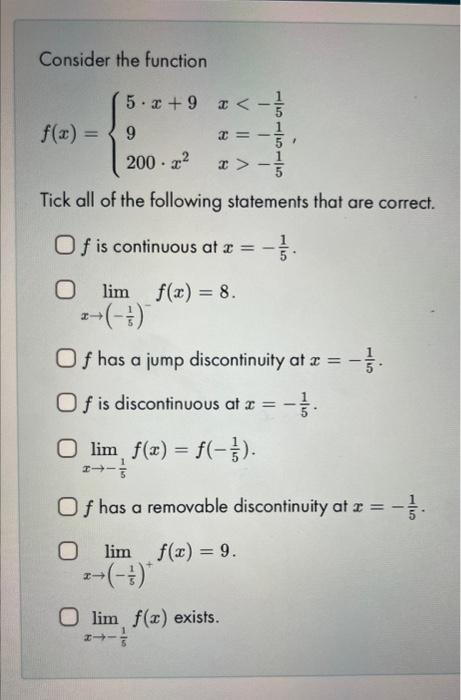Home / Expert Answers / Calculus / consider-the-function-f-x-left-begin-array-ll-5-cdot-x-9-x-frac-1-5-end-array-righ-pa837

# (Solved): Consider the function $f(x)=\left\{\begin{array}{ll} 5 \cdot x+9 & x-\frac{1}{5} \end{array}\righ ...Consider the function \[ f(x)=\left\{\begin{array}{ll} 5 \cdot x+9 & x<-\frac{1}{5} \\ 9 & x=-\frac{1}{5} \\ 200 \cdot x^{2} & x>-\frac{1}{5} \end{array}\right.$ Tick all of the following statements that are correct. $$f$$ is continuous at $$x=-\frac{1}{5}$$. $\lim _{x \rightarrow\left(-\frac{1}{5}\right)^{-}} f(x)=8$ $$f$$ has a jump discontinuity at $$x=-\frac{1}{5}$$. $$f$$ is discontinuous at $$x=-\frac{1}{5}$$. $\lim _{x \rightarrow-\frac{1}{5}} f(x)=f\left(-\frac{1}{5}\right) .$ $$f$$ has a removable discontinuity at $$x=-\frac{1}{5}$$. $\lim _{x \rightarrow\left(-\frac{1}{5}\right)^{+}} f(x)=9 .$ $$\lim _{x \rightarrow-\frac{1}{5}} f(x)$$ exists.

We have an Answer from Expert